# The OPTQP Procedure

### Example 12.2 Portfolio Optimization

Consider a portfolio optimization example. The two competing goals of investment are (1) long-term growth of capital and (2) low risk. A good portfolio grows steadily without wild fluctuations in value. The Markowitz model is an optimization model for balancing the return and risk of a portfolio. The decision variables are the amounts invested in each asset. The objective is to minimize the variance of the portfolio’s total return, subject to the constraints that (1) the expected growth of the portfolio reaches at least some target level and (2) you do not invest more capital than you have.

Let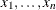be the amount invested in each asset,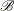be the amount of capital you have,be the random vector of asset returns over some period, andbe the expected value of. Let G be the minimum growth you hope to obtain, and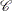be the covariance matrix of. The objective function is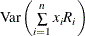, which can be equivalently denoted as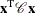.

Assume, for example, n = 4. Let= 10,000, G = 1000,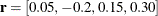, and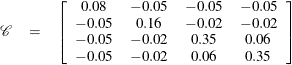The QP formulation can be written as follows: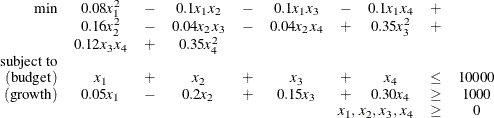The corresponding QPS-format input data set is as follows:

data portdata;
input field1 $field2$ field3 $field4 field5$ field6 @;
datalines;
NAME  .         PORT     .          .         .
ROWS  .         .        .          .         .
N     OBJ.FUNC  .        .          .         .
L     BUDGET    .        .          .         .
G     GROWTH    .        .          .         .
COLUMNS .       .        .          .         .
.     X1        BUDGET   1.0        GROWTH    0.05
.     X2        BUDGET   1.0        GROWTH    -.20
.     X3        BUDGET   1.0        GROWTH    0.15
.     X4        BUDGET   1.0        GROWTH    0.30
RHS   .         .        .          .         .
.     RHS       BUDGET   10000      .         .
.     RHS       GROWTH   1000       .         .
RANGES .        .        .          .         .
BOUNDS .        .        .          .         .
QUADOBJ .        .       .           .        .
.     X1        X1       0.16       .         .
.     X1        X2       -.10       .         .
.     X1        X3       -.10       .         .
.     X1        X4       -.10       .         .
.     X2        X2       0.32       .         .
.     X2        X3       -.04       .         .
.     X2        X4       -.04       .         .
.     X3        X3       0.70       .         .
.     X3        X4       0.12       .         .
.     X4        X4       0.70       .         .
ENDATA .        .        .          .         .
;


Use the following SAS statements to solve the problem:

proc optqp data=portdata
primalout = portpout
printlevel = 0
dualout   = portdout;
run;




The optimal solution is shown in Output 12.2.1.

Output 12.2.1: Portfolio Optimization

 The OPTQP Procedure Primal Solution

Obs Objective Function ID RHS ID Variable
Name
Variable
Type
Linear
Objective
Coefficient
Lower
Bound
Upper Bound Variable Value Variable
Status
1 OBJ.FUNC RHS X1 N 0 0 1.7977E308 3452.86 O
2 OBJ.FUNC RHS X2 N 0 0 1.7977E308 0.00 O
3 OBJ.FUNC RHS X3 N 0 0 1.7977E308 1068.81 O
4 OBJ.FUNC RHS X4 N 0 0 1.7977E308 2223.45 O

Thus, the minimum variance portfolio that earns an expected return of at least 10% is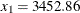,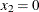,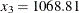,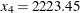. Asset 2 gets nothing, because its expected return is20% and its covariance with the other assets is not sufficiently negative for it to bring any diversification benefits. What if you drop the nonnegativity assumption? You need to update the BOUNDS section in the existing QPS-format data set to indicate that the decision variables are free.

   ...
RANGES .        .        .          .         .
BOUNDS .        .        .          .         .
FR    BND1      X1       .          .         .
FR    BND1      X2       .          .         .
FR    BND1      X3       .          .         .
FR    BND1      X4       .          .         .
QUADOBJ .        .       .          .         .
...


Financially, that means you are allowed to short-sell—that is, sell low-mean-return assets and use the proceeds to invest in high-mean-return assets. In other words, you put a negative portfolio weight in low-mean assets and more than 100% in high-mean assets. You can see in the optimal solution displayed in Output 12.2.2 that the decision variable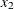, denoting Asset 2, is equal to1563.61, which means short sale of that asset.

Output 12.2.2: Portfolio Optimization with Short-Sale Option

 The OPTQP Procedure Primal Solution

Obs Objective Function ID RHS ID Variable
Name
Variable
Type
Linear
Objective
Coefficient
Lower Bound Upper Bound Variable Value Variable
Status
1 OBJ.FUNC RHS X1 F 0 -1.7977E308 1.7977E308 1684.35 O
2 OBJ.FUNC RHS X2 F 0 -1.7977E308 1.7977E308 -1563.61 O
3 OBJ.FUNC RHS X3 F 0 -1.7977E308 1.7977E308 682.51 O
4 OBJ.FUNC RHS X4 F 0 -1.7977E308 1.7977E308 1668.95 O